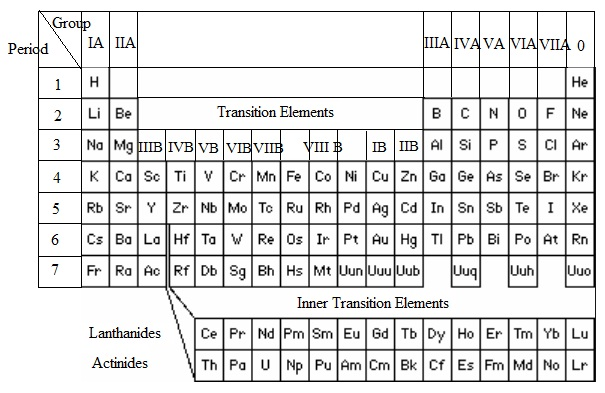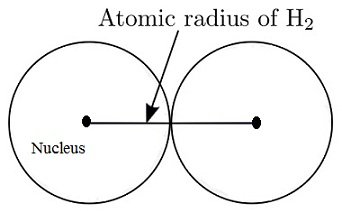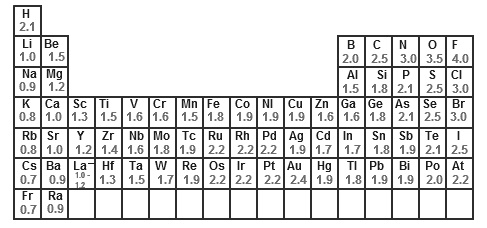#### Periodic Table-Gradations of Atomic properties, Chemistry tutorial

Introduction:

The periodic table comprises of elements arranged in groups and periods mainly based on their atomic number. The number of electrons in a neutral atom of an element provides the atomic number. The electrons occupy electronic shells. The elements in any period encompass the similar number of electronic shells and the number of valence electrons rises progressively through one across the period from left to right. Whenever elements are arranged in this manner, it was noticed that the properties of elements reappear at regular intervals. That is, the properties of the elements are a periodic function of their atomic number. The periodic properties give mount to vertical columns of groups or families of elements having the similar number of outer or valence elements. The elements in any group have closely associated physical and chemical properties. One great benefit of this is that it is only required to learn the properties of each and every group instead of the properties of each individual element.

Remember that the Bohr theory of shells was the base for the arrangement of electrons in atoms. Latest discoveries regarding the atoms have necessitated a revision of this idea. The electrons in atoms are now assumed to occupy areas in space around the nucleus termed as orbitals instead of fixed shells. Orbitals are simply areas in space around the nucleus where the probability of determining an electron is high; they are generally represented by s, p, d and f orbitals.

Brief Revision of the Electronic Configuration:

Electrons in atoms occupy different energy levels (n) and sub-energy levels (s, p, d, f - orbitals). Remember the Pauli's exclusion principle and Hund's rule in the arrangement of electrons in the energy levels. The summary beneath will revive your memory.Fig: Electronic Configuration

For instance, sodium by atomic number 11 will encompass the electronic structure 1s2 2s2 2p6 3s1; magnesium having atomic number 1s2 2s2 2p6 3s2 and so forth.

Electronic Configuration and the Periodic Table:

The physical and chemical properties of an element are controlled by the number and arrangement of the orbital electrons, that is, via the atomic number. The modern periodic classification (that is, The Periodic Table) groups atoms of the element according to their electronic configurations.

Elements having one 's' electron in their outer shell are termed as Group I (that is, the alkali metals) and elements having two 's'  electrons in their outer shell are termed as Group H (that is, the alkaline earth metals). Such two groups are termed as the 's' block elements, as their properties result from the presence of 's' electrons. Elements having three electrons in their outer shell (that is, two 's' electrons and one 'p' electron) are termed as Group III, and likewise Group IV elements contain four outer electrons, Group V elements contain five outer electrons, Group VI elements comprise of six outer electrons and Group VII elements encompass seven outer electrons. Group 0 elements encompass a full outer shell of electrons in such a way that the next shell is empty; therefore the group name. Groups III, IV, V, VI, VII and 0 all encompass 'p' orbitals filled and because their properties are based on the presence of 'p' electrons, they are termed as jointly the 'p' block elements.

In a similar manner, elements where 'd' orbitals are being filled are known as the 'd' block, or transition elements. In such, 'd' electrons are being added to the penultimate shell (that is, one shell before the outer shell). Lastly, elements where 'f'  orbitals are filling are termed as the 'f' block, and here the 'f' electrons are entering the antepenultimate (or second shell from the outer shell) shell.

The summary of block arrangement of elements mainly based on the outermost energy levels for s- and p- block elements; and the orbitals being filled for d- and f- block elements.

Group I: One s electron [s-block]

Group II: Two s electrons [s-block]

Group III: Two s electrons + One p electron [p-block]

Group IV: Two s electrons + Two p electrons [p-block]

Group V: Two s electrons + Three p electrons [p-block]

Group VI: Two s electrons + Four p electrons [p-block]

Group VII: Two s electrons + Five p electrons [p-block]

Group 0: Two s electrons + Six p electrons [p-block]

Transition Elements:  d-orbitals being filled by electrons - d-block Inner Transition Elements: f-orbitals being filled with electrons - f- block (Lanthanides and Actinides)Fig: Periodic table

In the periodic table (table shown above) the elements are arranged in order of increasing atomic number, that is, in order of increased nuclear charge, so that each and every element has one more orbital electron than the prior element. Rather than listing the 103 elements, the periodic table arranges them to some rows or periods, in such a manner that each and every row starts with an alkali metal and ends by an inert gas. The sequence in which the different energy levels are filled finds out the number of elements in each and every period, and the periodic table can be divided into four major areas according to whether the s, p, d or f levels are being filled.

1st period   1s             elements in this period = 2

2nd period  2s 2p        elements in this period = 8

3rd period   3s 3p        elements in this period = 8

4th period   4s 3d 4p   elements in this period = 18

5th period   5s 4d 5p   elements in this period = 18

6th period   6s 5d 6p   elements in this period = 32

This form of the periodic table consists of many merits. The similarity of properties in a group and the relation between the group and the electron structure is highlighted. The d-block elements are termed to as the transition elements as they are positioned between the s and p blocks. Hydrogen and helium distinct from the rest of the elements as there are no 'p' orbitals in the first shell. Helium perceptibly belongs to Group 0, the inert gases, that are chemically inactive as their outer shell of electrons is full. Hydrogen is more complex to place in a group. This could be included in Group I because it consists of one 's' electron in its outer shell, or in Group VII as it is one electron short of a complete shell. Hydrogen is comprised in both these groups in the periodic table; however it looks like neither the alkali metals nor the halogens very closely. The exceptional properties of hydrogen are largely due to the extremely small size of hydrogen atoms. Therefore, there is a case for positioning hydrogen in a group on its own, or omitting it from the periodic table altogether.

The Periodic Table and Atomic Properties:

We know that chemical and physical properties of the elements differ periodically by the atomic number. The periodic table thus gives an organized structure to the knowledge and understanding the chemistry of the elements. Except from this, there is as well a variation of atomic properties of elements in the periodic table. A few of these properties are atomic and ionic sizes, ionization energy, electron affinity and electronegativity.

Atomic size:

The atom is an extremely small entity and therefore it is difficult to find out its size that is, distance from the center of the nucleus to the outermost orbital. Though by the help of modem methodologies like X-ray and electron diffraction, it is possible to find out the distance between covalently bonded atoms. For illustration: the distance between the nuclei of oxygen atoms in the oxygen molecule is 0.132 nm, therefore the atomic radius of an oxygen atom is around 0.066 nm. The atomic radius or sizes of any atom is taken to be one-half the distance of closest approach between the nuclei of atoms in the elemental substance.The atomic size reduces from left to right across a period in the periodic table. In another words, as the atomic number rises across any period, the size of the atom decreases. Remember that as we move across a period one electron is added rousingly from one element to the subsequent and the electrons are being added to the similar shell at about the similar distance from the nucleus. At similar time, protons are as well being added to the nucleus. Rise in the number of proton, increases the nuclear charge that progressively apply a stronger attraction on the electrons around it and would pull them towards the nuclei. As the nuclear charge increases having atomic number across a period, the attractive force applied by the nucleus on the outermost electrons of the atom increases therefore the atomic radius or size reduces across a period. For illustration: on moving from Lithium to beryllium, the number of charges on the nucleus is increased by one, thus all the orbital electrons are pulled in closer to the nucleus. In a particular period, the alkali, metal is the largest atom and the halogen is the smallest.

The ions of elements are made up whenever atoms lose or gain electron. The size of an ion is termed as ionic radii are different from atomic sizes. Ionic sizes are computed in electrovalent compounds.

The ionic radius of a specific compound is the distance between the centre of one ion and the centre of its nearby neighbor of the opposite charge.

A positive ion is made up by eliminating one or more electrons from an atom. Whenever this takes place, the number of positive nuclear charge is more than the number of negative electronic charge; therefore the electrons are pulled in. A positive ion is thus smaller than the corresponding atom and the more electrons eliminated (i.e., the greater the charge on the ion), the smaller it becomes example:

Atomic radius Na = 0.186 nm   Atomic radius Fe = 0.139 nm

Whenever a negative ion is made up, one or more electrons are added to an atom. The number of positive nuclear charge is now less than the number of negative electronic charges therefore the pull on the electrons is decreased.

In common, ionic radii of negative ions are more than the corresponding atomic radii that is, negative ions are bigger than the corresponding atom example:

Atomic radius of Cl = 0.099

Ionic radius of Cl = 0.128

Ionization energy:

Whenever energy is supplied to an atom, electrons might be promoted to a higher energy level. If sufficient energy is supplied, the electron might be fully eliminated, giving a positive ion. The energy needed to eliminate the most loosely bound electron from each atom in a mole of gaseous atom, generating one mole of gaseous ion is termed as ionization energy (KJ mol-1). As it is possible to eliminate one, two or three.... electrons from most atoms there is first second or third... ionization energy (I.E.).

M   →     M+   →    M2+   →     M3+    →

1st I.E.       2nd I.E.     3rd I.E.

The factors affecting the ionization energy are the:

i) Size of the atom that is a measure of the outermost electron from the nucleus.

ii) Charge on the nucleus - as nuclear charge rises, the attraction for outermost electron increases, and so more energy is needed to ionize.

iii) Screening effect of the inner electron shells - the outermost electrons are repelled via all the other inner shell electrons and prevented from experiencing the full attraction of the positively charged nucleus:

Across a period, the first ionization energy rises as atomic number rises as the atomic radius reduces. As the distance decreases, the attraction of the positive nucleus for the electron will increase; therefore more energy is needed to get rid of the outermost electron therefore the ionization energy will increase.

It will be noted that the screening effect remain almost similar across a period as electrons are added to the similar shell. The table below represents the first ionization energies of the first twenty elements.

 1 2 3 4 5 6 7 0 1 H 1311 He 2372 2 Li 520 Be 899 B 801 C 1086 N 1403 O 1410 F 1681 Ne 2080 3 Na 496 Mg 737 Al 577 Si 786 P 1012 S 999 Cl 1255 Ar 1521 4 K 419 Ca 590

Fig: First Ionization Energies of the Elements (KJ mol-1)

The first ionization energies of the elements in the first two short periods are illustrated in the figure above. These illustrate a general upward trend from Li to Ne and from Na to Ar. The values for Ne and Ar are the highest in their periods as it needs a great deal of energy to break a stable filled shell of electrons. There are some irregularities. The high values for Be and Mg are featured to the stability of a filled 's' level. The high values for N and P point out that a half-filled 'p' level is as well specifically stable. The values for B and Al are lower because elimination of one electron leaves a stable filled 's' shell, and likewise with 0 and S a stable half-filled 'p' shell is left.

Filled s level example: Be 1s2 2s2

Mg 1s2 2s2 2p6 3s2

Half-filled p level example: N 1s2 2s2 2p3

P 1s2 2s2 2p6 3s2 3p3

Completely full - inert gas structure

Example: Nc 1s2 2s2 2p6

Ar 1s2 2s2 2p6 3s2 3p6

In common, the ionization energy decreases on descending a group because an additional shell of electron is added that as well help to screen the outer electron from the nucleus. This trend is illustrated by the alkali, metals from Li (520) to Na (496) to K (419).

Electron affinity:

Electron affinity has to do by the gain of electrons to form negative ions whereas ionization deals by loss of electrons to form positive ions.  The energy discharged if an additional electron is added to a neutral gaseous atom to make a univalent negative ion is known as the electron affinity.  As energy is given off in the process, electron affinity consists of a negative value. Electron affinities based on the size and efficient nuclear charge of the atom. Moving from left to right across a period, electron affinity reduces (that is, increase in negative value), anticipate for the noble gases that encompass positive values. Down a group of the periodic table, electron affinities increase (that is, decrease in negative value). The reason for the noticed trend is that atoms having smaller atomic radii tend to encompass a stronger attraction for electrons and therefore form negative ions more readily.

Element           Energy (kJ mol-1 negative value)

Li → Li                      57

Na → Na-                  21

Al → Al-                     26

P → P-                       60

S → S-                       200

Cl → Cl-                     348

Electronegativity:

The tendency of an atom in a molecule to attract the bonded electrons to itself is known as the electronegativity of the atom.  Usually, small atoms attract electrons (that is, due to closeness of the nucleus) more than large ones and are thus more electronegative. Atoms having nearly filled shells of electrons will tend to encompass higher electronegativity (due to the desire to encompass a stable filled shell) than those having sparsely occupied shells.

The electronegativity of elements decrease down a group and increase across the period.  The reason for the trend is that down the group, atomic size rises and effective nuclear charge decreases therefore electron attracting power (that is, electronegativity) of the atom decreases. From left to right of a period, the opposite effect is noticed, atomic size reduces and efficient nuclear charge increases, these join to increase the electronegativity. The most electronegative elements are the reactive non-metals (example: Fluorine) at the top right-hand corner of the periodic table whereas the least electronegative elements are the reactive metals (example:  caesium) at the bottom left-hand corner of the period table.Table: Electronegativity volume

Tutorsglobe: A way to secure high grade in your curriculum (Online Tutoring)